# Heptagonal tiling

Heptagonal tiling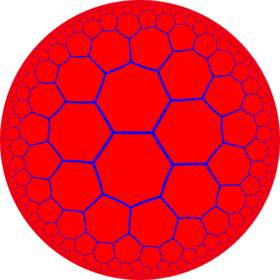Poincaré disk model of the hyperbolic plane
TypeHyperbolic regular tiling
Vertex figure73
Schläfli symbol{7,3}
Wythoff symbol3 | 7 2
Coxeter diagramSymmetry group[7,3], (*732)
DualOrder-7 triangular tiling
PropertiesVertex-transitive, edge-transitive, face-transitive

In geometry, the heptagonal tiling is a regular tiling of the hyperbolic plane. It is represented by Schläfli symbol of {7,3}, having three regular heptagons around each vertex.

## Images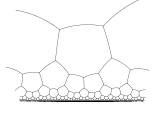Poincaré half-plane model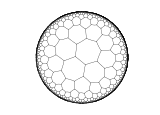Poincaré disk model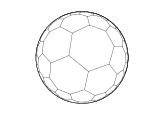Klein-Beltrami model

This tiling is topologically related as a part of sequence of regular polyhedra with Schläfli symbol {n,3}.

From a Wythoff construction there are eight hyperbolic uniform tilings that can be based from the regular heptagonal tiling.

Drawing the tiles colored as red on the original faces, yellow at the original vertices, and blue along the original edges, there are 8 forms.

## Hurwitz surfaces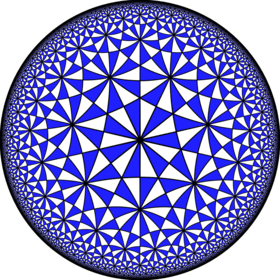The symmetry group of the heptagonal tiling has fundamental domain the (2,3,7) Schwarz triangle, which yields this tiling.
Further information: Hurwitz surface

The symmetry group of the tiling is the (2,3,7) triangle group, and a fundamental domain for this action is the (2,3,7) Schwarz triangle. This is the smallest hyperbolic Schwarz triangle, and thus, by the proof of Hurwitz's automorphisms theorem, the tiling is the universal tiling that covers all Hurwitz surfaces (the Riemann surfaces with maximal symmetry group), giving them a tiling by heptagons whose symmetry group equals their automorphism group as Riemann surfaces. The smallest Hurwitz surface is the Klein quartic (genus 3, automorphism group of order 168), and the induced tiling has 24 heptagons, meeting at 56 vertices.

The dual order-7 triangular tiling has the same symmetry group, and thus yields triangulations of Hurwitz surfaces.Wikimedia Commons has media related to Order-3 heptagonal tiling.